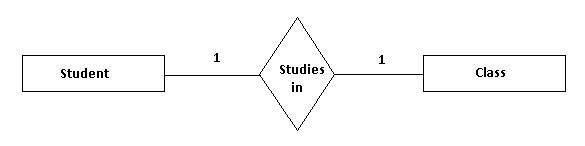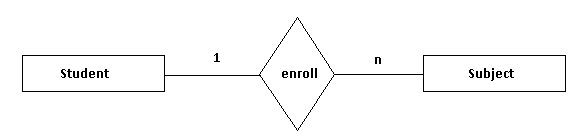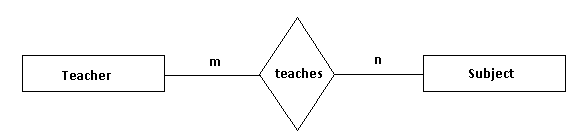## E-R Model

Entity Relationship model is a technique that helps us model real-world systems in the form of software constructs. E-R Model defines the conceptual view of database. E-R diagram is a visual way used to represent data and how data is related to each other.

#### Types of Relationships

In E-R model, relationships can be classified into three types:1. One-to-One: In one-to-one (1:1) relationship, for a given occurrence of an entity (i.e. its value), there is exactly one occurrence of another entity. For example, a student can study in one class at a time.2. One-to-Many: In one-to-many (1:n) relationship, for a given occurrence of an entity (i.e. its value), there can be one or more occurrences of another entity. A student can enroll to many subjects.3. Many-to-Many: In many-to-many (m:n) relationship, there can be one or more values on both sides of a relationship. It means there can be multiple instances of both entities. For example, a teacher can teach multiple subjects, similarly a subject can have multiple teachers assigned to it.« Back to Course page